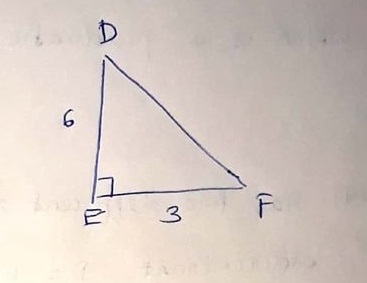# Find m\angle F FSP19611001661.jpgFSZJosh Sizemore 2021-12-14 Answered
Find $m\mathrm{\angle }F$You can still ask an expert for help

• Live experts 24/7
• Questions are typically answered in as fast as 30 minutes
• Personalized clear answers

Solve your problem for the price of one coffee

• Math expert for every subject
• Pay only if we can solve itVivian Soares
Step 1
Given,
a right angle triangle
$\mathrm{\angle }E={90}^{\circ }$
$ED=6$
$EF=3$
Step 2
Let $\mathrm{\angle }F=\theta$
then $\mathrm{tan}\theta =\frac{p}{b}=\frac{DE}{EF}$
$⇒\mathrm{tan}\theta =\frac{6}{3}$
$⇒\mathrm{tan}\theta =2$
$⇒\theta ={\mathrm{tan}}^{-1}2$
$⇒\theta =63.434$
$\stackrel{\sim }{=}63.4$

We have step-by-step solutions for your answer!Ethan Sanders

Step 1
$b=6$
$c=3$
$\alpha ={90}^{\circ }$
Step 2
${a}^{2}={b}^{2}+{c}^{2}-2bc\mathrm{cos}\alpha$
$a=\sqrt{{b}^{2}+{c}^{2}-2bc\mathrm{cos}\alpha }$
$a=\sqrt{{6}^{2}+{3}^{2}-2×6×3×{\mathrm{cos}90}^{\circ }}$
$a=6.71$
Step 3
$a=6.71$
$b=6$
$c=30$
${a}^{2}={b}^{2}+{c}^{2}-2bc\mathrm{cos}\alpha$
$\alpha =\mathrm{arccos}\left(\frac{{b}^{2}+{c}^{2}-{a}^{2}}{2bc}\right)=\mathrm{arccos}\left(\frac{{6}^{2}+{3}^{2}-{6.71}^{2}}{2×6×3}\right)={90}^{\circ }$
${b}^{2}={a}^{2}+{c}^{2}-2ac\mathrm{cos}\beta$
$\beta =\mathrm{arccos}\left(\frac{{a}^{2}+{c}^{2}-{b}^{2}}{2ac}\right)=\mathrm{arccos}\left(\frac{{6.71}^{2}+{3}^{2}-{6}^{2}}{2×6.71×3}\right)={63}^{\circ }$
Answer: $m\mathrm{\angle }F={63}^{\circ }$

We have step-by-step solutions for your answer!